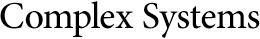Sequences Generated by Neuronal Automata with Memory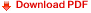Michel Cosnard
Laboratoire LIP-IMAG/CNRS, Ecole Normale Supérieure de Lyon,
69364 Lyon Cedex 07, France

Maurice Tchuente
Gilbert Tindo
Département d'Informatique,
Faculté des Sciences, BP 812 Yaoundé, Cameroun

Abstract

We study the sequences generated by neuronal recurrence equations of the form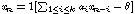, where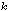is called the memory length. We show that, if there is a neuronal recurrence equation with memory lengththat generates sequences of periods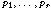, then there is a neuronal recurrence equation with memory length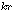that generates a sequence of period lcm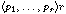, where lcm denotes the least common multiple. As an application we show that, for any integer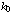, there is a neuronal equation with memory length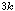,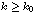, that generates a sequence of period.